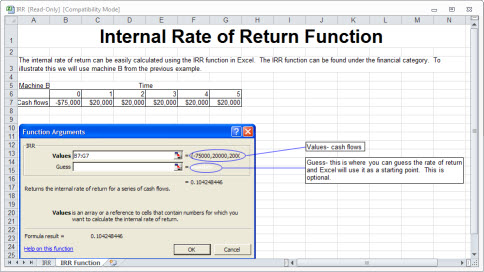## Answered By: Peter Z McKay Last Updated: Oct 29, 2014     Views: 855

• INTERNAL RATE OF RETURN (IRR)
StudyFinance.com

The internal rate of return is simply the rate of return on an investment.  IRR is similar to the  net present value calculation.  The NPV calculation finds the net present value using a predefined discount rate.  IRR finds the discount rate that makes the NPV equal to zero.  The discount rate is the cost of borrowing or using money for investments.  The decision to accept or reject the purchase depends on the whether the internal rate of return is higher than the discount rate. The decision criteria for these projects is simple, accept the project if the IRR is higher than the discount rate or the cost of borrowing.  There are also financing decisions, where there are cash inflows followed by cash outflows.  For example, magazine publishers recieve subscription payments in the beginning and then incur delivery costs later.  In the case of financing projects the decision criteria is opposite to that of the investing projects, accept the financing project if the IRR is less than the discount rate or cost of borrowing.  In the magazine example accept the project if it is cheaper to finance or borrow the money from the subscribers than the bank.